# 数据结构与算法—最小生成树(Prim算法和Kruskal算法算法详解)

### 前言

##### 一个故事

• 初始，各个城市没有高速公路(铁路)。城市没有！
• 政府打算各个城市铺设公路(铁路)，每个城市都想成为交通枢纽，快速到达其他城市！但是这种情况下国家集体资源跟不上、造价太昂贵。并且造成巨大浪费！
• 最终国家选择一些主要城市进行联通，有个别城市只能稍微绕道而行，而绕道太远的、人流量多的国家考虑新建公路(铁路)。适当提高效率。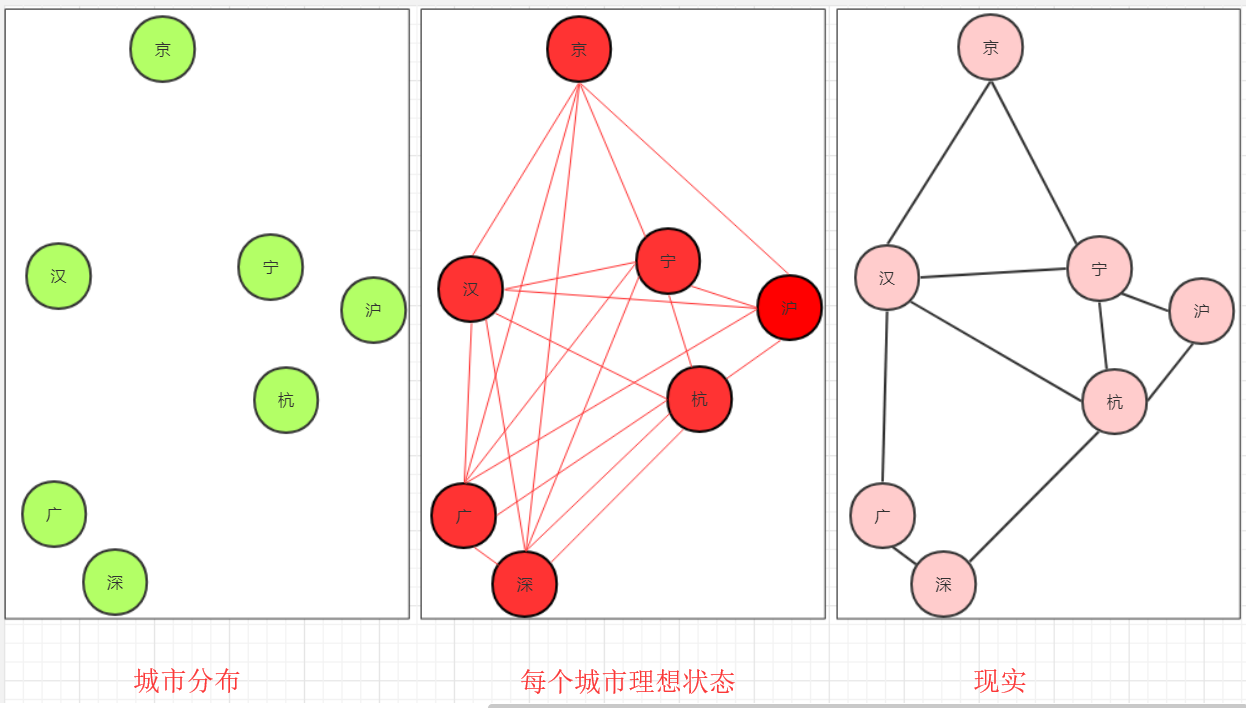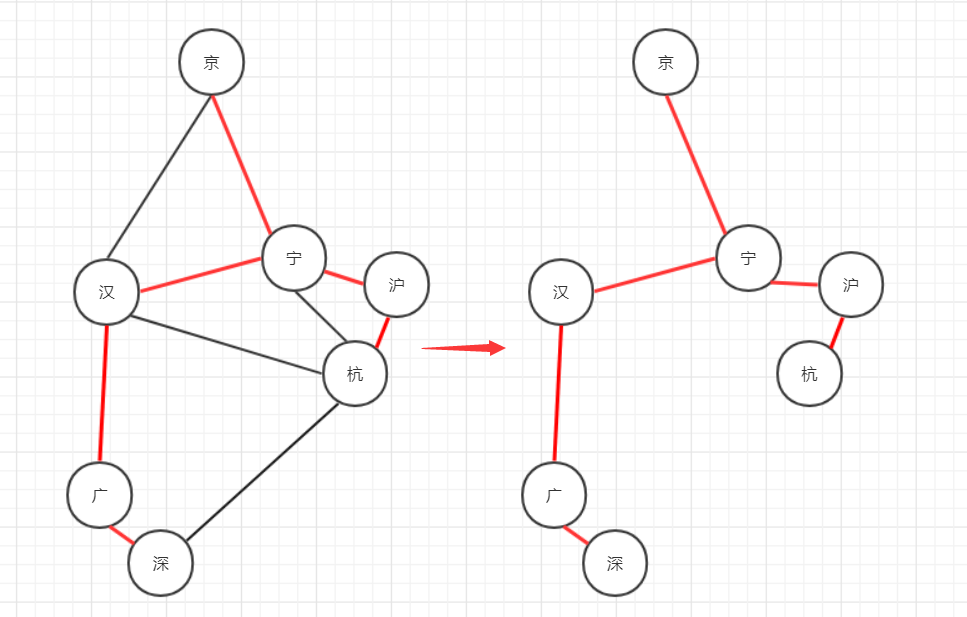• 问题的主要矛盾对问题起着决定性作用。主要矛盾次要矛盾相互影响，相互渗透，一定程度可以相互转化。故我们看问题要抓关键、找核心
• 公路时代城市联通的主要矛盾是时间慢，而造价相比运输时间是次要矛盾。所以在公路时代我们尽量使得城市能够直接联通，缩短城市联系时间。而稍微考虑建路成本！随着科技发展、信息传输相比公路运输很快，从而事件的主要矛盾从运输时间转变为造价成本。所以我们会关注联通所有点的路程(最短)。这就用到最小生成树算法。

### Kruskal算法

1. 将边(以及2顶点)的对象依次加入集合(优先队列)q1中。初始所有点相互独立
2. 取出当前q1最小边，判断边的两点是否联通。
3. 如果联通，跳过，如果不连通，则使用union（并查集合并）将两个顶点合并。这条边被使用(可以储存或者计算数值)。
4. 重复2，3操作直到集合（优先队列）q1为空。此时被选择的边构成最小生成树。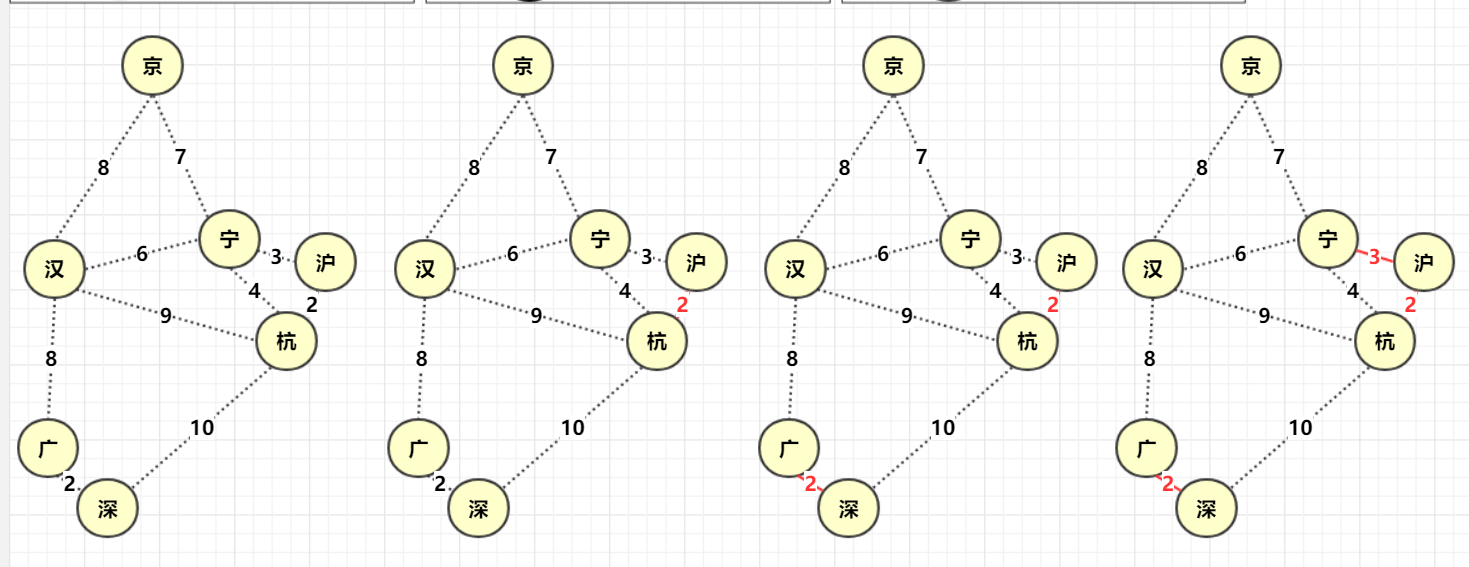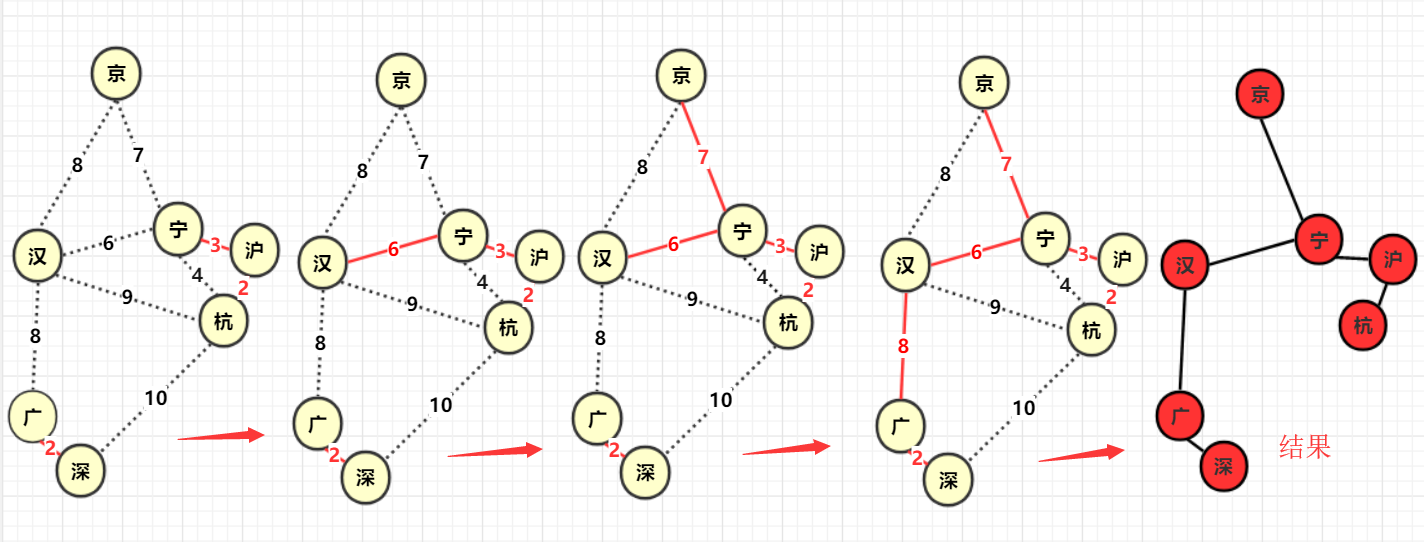### Prim算法

1. 寻找图中任意点，以它为起点，它的所有边V加入集合(优先队列)q1,设置一个boolean数组bool[]标记该位置已经确定。
2. 从集合q1找到距离最小的那个边v1判断边另一点p是否被标记(访问)，如果p被标记说明已经确定那么跳过，如果未被标(访问)记那么标记该点p,并且与p相连的未知点(未被标记)构成的边加入集合q1边v1(可以进行计算距离之类，该边构成最小生成树) .
3. 重复1，2直到q1为空，构成最小生成树 ！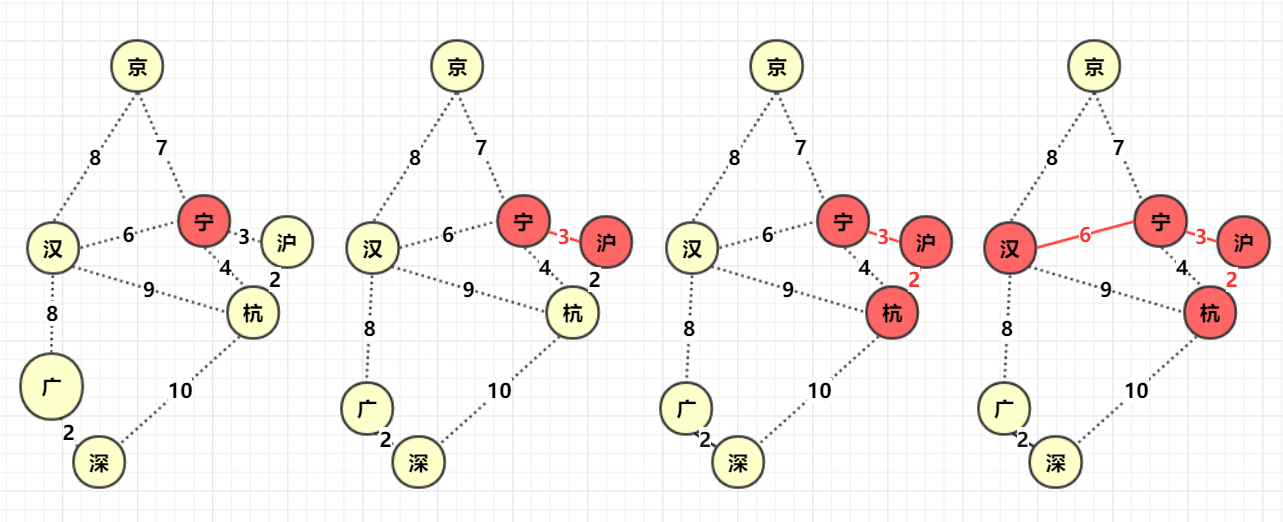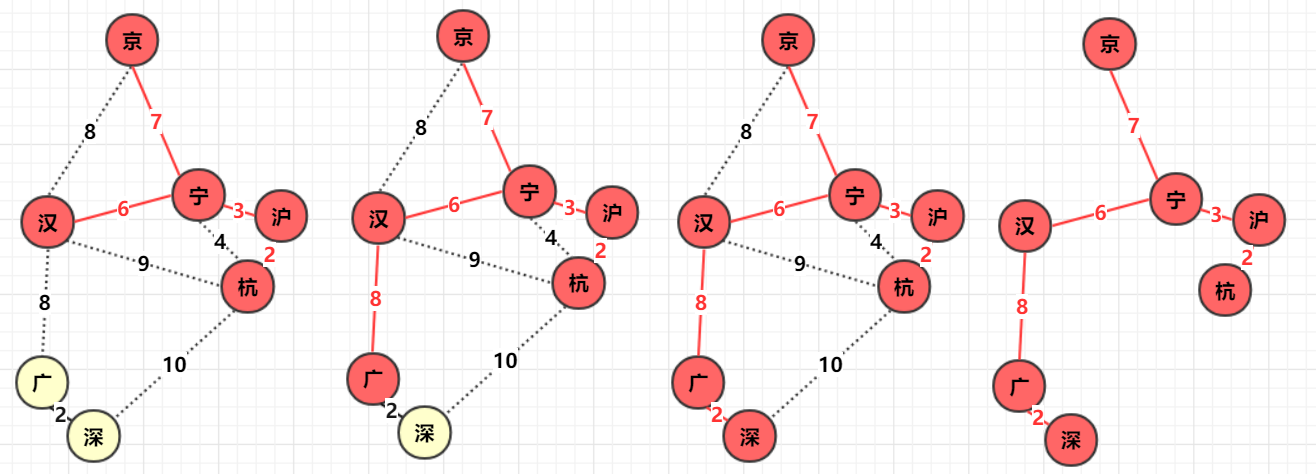• 能够保证所有节点连通(能够满足要求和条件)
• 能够保证所有路径之和最小(结果和目的相同)
• 最小生成树不唯一，可能多样的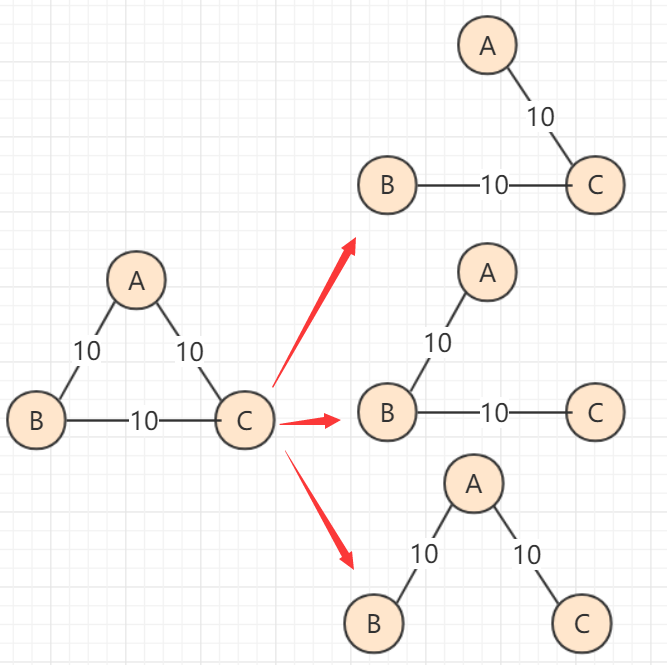### 代码实现

###### prim
package 图论;

import java.util.ArrayList;
import java.util.Arrays;
import java.util.Comparator;
import java.util.List;
import java.util.PriorityQueue;
import java.util.Queue;

public class prim {

public static void main(String[] args) {
int minlength=0;//最小生成树的最短路径长度
int max=66666;
String cityname[]= {"北京","武汉","南京","上海","杭州","广州","深圳"};
int city[][]= {
{ max, 8, 7, max, max, max, max }, //北京和武汉南京联通
{ 8, max,6, max,9, 8,max }, //武汉——北京、南京、杭州、广州
{ 7, 6, max, 3,4, max,max }, //南京——北京、武汉、上海、杭州
{ max, max,3, max,2, max,max }, //上海——南京、杭州
{ max, 9,4, 2,max, max,10 }, //杭州——武汉、南京、上海、深圳
{ max, 8,max, max,max, max,2 }, //广州——武汉、深圳
{ max, max,max, max,10,2,max }//深圳——杭州、广州
};// 地图

boolean istrue[]=new boolean;
//南京
Queue<side>q1=new PriorityQueue<side>(new Comparator<side>() {
public int compare(side o1, side o2) {
// TODO Auto-generated method stub
return o1.lenth-o2.lenth;
}
});
for(int i=0;i<7;i++)
{
if(city[i]!=max)
{
istrue=true;
}
}
while(!q1.isEmpty())
{
side newside=q1.poll();//抛出
if(istrue[newside.point1]&&istrue[newside.point2])
{
continue;
}
else {
if(!istrue[newside.point1])
{
istrue[newside.point1]=true;
minlength+=city[newside.point1][newside.point2];
System.out.println(cityname[newside.point1]+" "+cityname[newside.point2]+" 联通");
for(int i=0;i<7;i++)
{
if(!istrue[i])
{
}
}
}
else {
istrue[newside.point2]=true;
minlength+=city[newside.point1][newside.point2];
System.out.println(cityname[newside.point2]+" "+cityname[newside.point1]+" 联通");
for(int i=0;i<7;i++)
{
if(!istrue[i])
{
}
}
}
}

}
System.out.println(minlength);
}

static class side//边
{
int lenth;
int point1;
int point2;
public side(int lenth,int p1,int p2) {
this.lenth=lenth;
this.point1=p1;
this.point2=p2;
}
}

}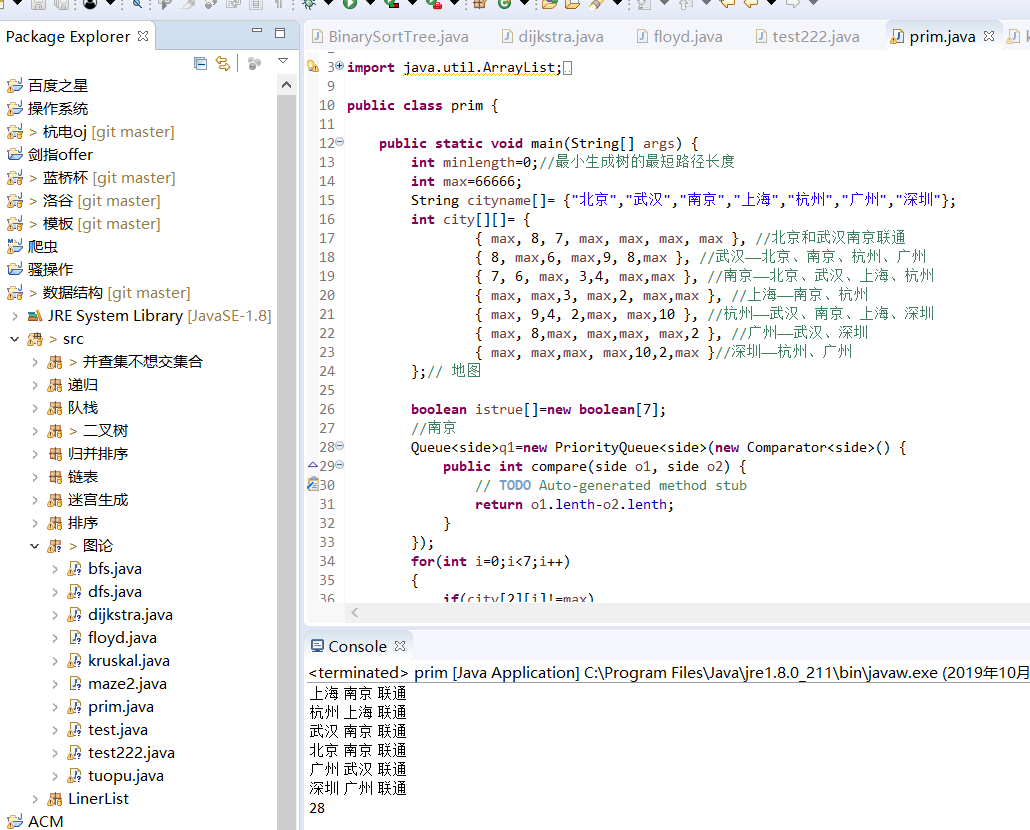###### Kruskal:
package 图论;

import java.util.Comparator;
import java.util.PriorityQueue;
import java.util.Queue;

import 图论.prim.side;
/*
* 作者：bigsai(公众号)
*/
public class kruskal {

static int tree[]=new int;//bing查集
public static void init() {
for(int i=0;i<10;i++)//初始
{
tree[i]=-1;
}
}
public static int search(int a)//返回头节点的数值
{
if(tree[a]>0)//说明是子节点
{
return tree[a]=search(tree[a]);//路径压缩
}
else
return a;
}
public static void union(int a,int b)//表示 a，b所在的树合并小树合并大树(不重要)
{
int a1=search(a);//a根
int b1=search(b);//b根
if(a1==b1) {//System.out.println(a+"和"+b+"已经在一棵树上");
}
else {
if(tree[a1]<tree[b1])//这个是负数，为了简单减少计算，不在调用value函数
{
tree[a1]+=tree[b1];//个数相加  注意是负数相加
tree[b1]=a1;       //b树成为a的子树，直接指向a；
}
else
{
tree[b1]+=tree[a1];//个数相加  注意是负数相加
tree[a1]=b1;       //b树成为a的子树，直接指向a；
}
}
}
public static void main(String[] args) {
// TODO Auto-generated method stub
init();
int minlength=0;//最小生成树的最短路径长度
int max=66666;
String cityname[]= {"北京","武汉","南京","上海","杭州","广州","深圳"};
boolean jud[][]=new boolean;//加入边需要防止重复 比如 ba和ab等价的
int city[][]= {
{ max, 8, 7, max, max, max, max },
{ 8, max,6, max,9, 8,max },
{ 7, 6, max, 3,4, max,max },
{ max, max,3, max,2, max,max },
{ max, 9,4, 2,max, max,10 },
{ max, 8,max, max,max, max,2 },
{ max, max,max, max,10,2,max }
};// 地图
boolean istrue[]=new boolean;
//南京
Queue<side>q1=new PriorityQueue<side>(new Comparator<side>() {//优先队列存边+
public int compare(side o1, side o2) {
// TODO Auto-generated method stub
return o1.lenth-o2.lenth;
}
});
for(int i=0;i<7;i++)
{
for(int j=0;j<7;j++)
{
if(!jud[i][j]&&city[i][j]!=max)//是否加入队列
{
jud[i][j]=true;jud[j][i]=true;
}
}
}
while(!q1.isEmpty())//执行算法
{
side newside=q1.poll();
int p1=newside.point1;
int p2=newside.point2;
if(search(p1)!=search(p2))
{
union(p1, p2);
System.out.println(cityname[p1]+" "+cityname[p2]+" 联通");
minlength+=newside.lenth;
}
}
System.out.println(minlength);

}
static class side//边
{
int lenth;
int point1;
int point2;
public side(int lenth,int p1,int p2) {
this.lenth=lenth;
this.point1=p1;
this.point2=p2;
}
}
}


###### kruskal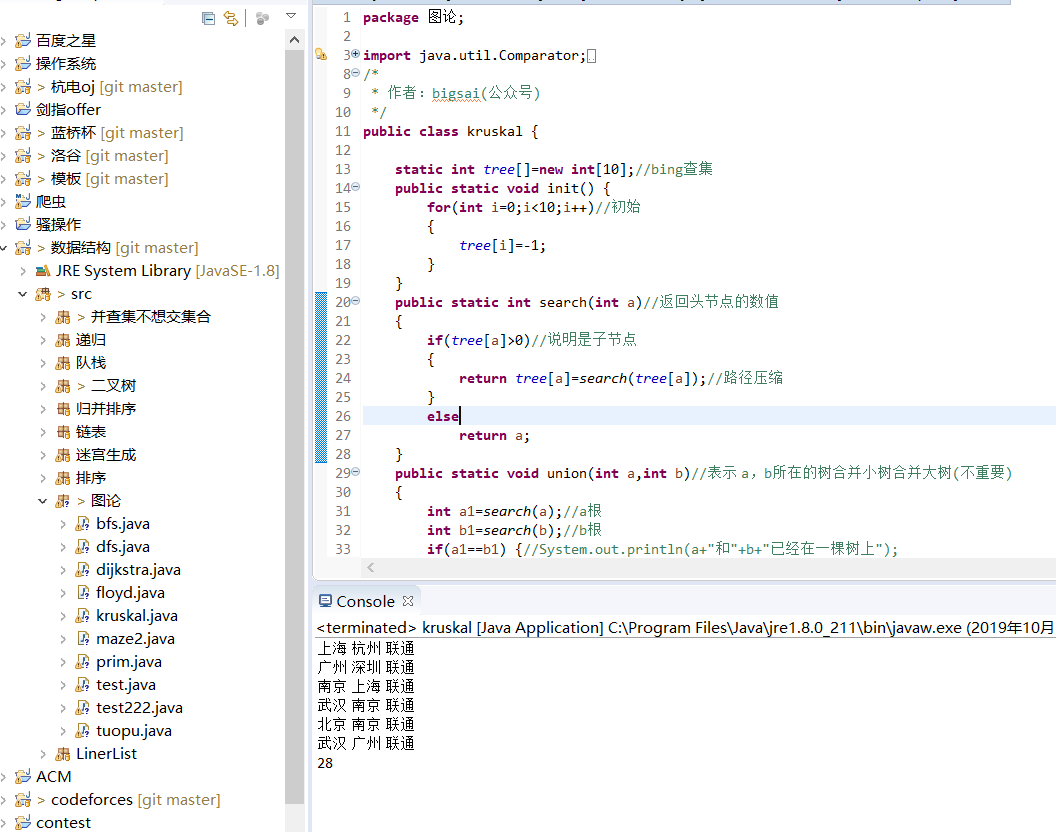### 总结©️2019 CSDN 皮肤主题: 代码科技 设计师: Amelia_0503